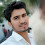# HackerRank Java BigDecimal problem solution

In this HackerRank Java BigDecimal problem in the java programming language Java's BigDecimal class can handle arbitrary-precision signed decimal numbers. Let's test your knowledge of them!

Given an array, s, of n real number strings, sort them in descending order — but wait, there's more! Each number must be printed in the exact same format as it was read from stdin, meaning that .1 is printed as .1, and 0.1 is printed as 0.1. If two numbers represent numerically equivalent values (e.g., .1 = 0.1), then they must be listed in the same order as they were received as input).

## HackerRank Java BigDecimal problem solution.

```import java.math.BigDecimal;
import java.util.*;
class Solution{

public static void main(String []args){
//Input
Scanner sc= new Scanner(System.in);
int n=sc.nextInt();
String []s=new String[n+2];
for(int i=0;i<n;i++){
s[i]=sc.next();
}
sc.close();

for(int i=1; i<n ; i++){
for(int j=i; j>=1; j--){

if(new BigDecimal(s[j]).compareTo(new BigDecimal(s[j-1]))>0){
String temp = s[j];
s[j] = s[j-1];
s[j-1] = temp;

}else{
break;
}
}
}

//Output
for(int i=0;i<n;i++)
{
System.out.println(s[i]);
}
}

}```

## Second solution in java 8 programming

```import java.io.BufferedReader;
import java.io.IOException;
import java.math.BigDecimal;
import java.util.Arrays;
import java.util.Comparator;

public class Solution
{
public static class V {
public final String s;
public final BigDecimal b;

public V(final String s) {
this.s = s;
this.b = new BigDecimal(s);
}
}

public static void main(String[] args) throws IOException {

final int N = Integer.parseInt(br.readLine().trim(), 10);

final V[] A = new V[N];
for (int i = 0; i < N; i++) {
}
Arrays.sort(A, new Comparator<V>() {
@Override
public int compare(final V o1, final V o2) {
return o1.b.compareTo(o2.b);
}
});
final StringBuilder sb = new StringBuilder();
for (int i = N - 1; i >= 0; i--) {
sb.append(A[i].s).append('\n');
}

System.out.print(sb.toString());

br.close();
br = null;
}
}```

1.